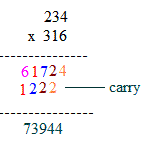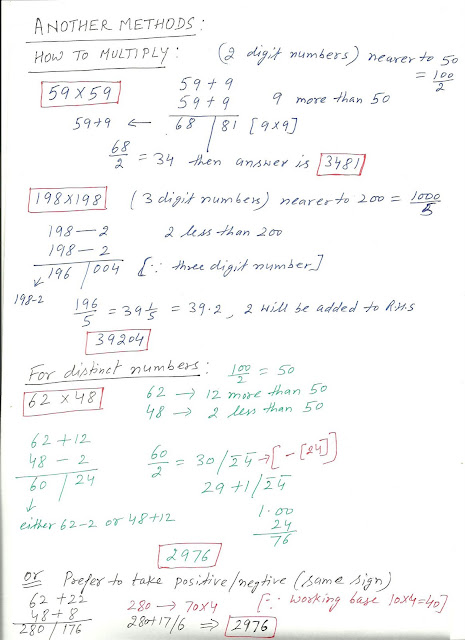## Friday, June 8, 2012

IT IS THE ATTITUDE AND NOT THE APTITUDE WHICH DETERMINES THE ALTITUDE.

SUCCESS IS NOT COME TO THE PERSON IT IS TO BE ACHIEVED.

THERE IS ENOUGH SPACE IN THIS UNIVERSE BUT ONE HAS TO CREATE IT FOR ONESELF.

Vedic Mathematics is the name given to the ancient system of Mathematics which was rediscovered from the Vedas between 1911 and 1918 by Sri Bharati Krsna Tirthaji (1884-1960). At the beginning of the twentieth century, when there was a great interest in the Sanskrit texts in Europe, Bharati Krsna Swamiji tells us some scholars ridiculed certain texts which were headed 'Ganita Sutras'- which means mathematics.

1. High Speed Vedic Mathematics is 10-15 times faster than normal Maths

2. Better and Much Improved Academic Performance in school and Instant Results

3. Sharpens your mind, increases mental agility and intelligence

4. A Complete System comprising all the benefits of Mental Maths .

•  Learn how to multiply numbers through easier mathods for an examples

• 65X65=4225 , 95X95=9025, 105X105=11025(6x7=42 and write square of 5 i.e., 25 then it becomes 4225 similarly for those numbers who ends with 5)
111X111=12321 (within seconds) many more multiplications like 679x567=  ............﻿ ﻿ by following method .I have used sargam for indicating multiplication like sa(vertical),re(cross), ga(cross then vertical) as given below:

For two digit numbers , sa (vertical multiplication) , re (cross) then apply sa.There are 3 steps:
a) Multiply vertically on the left: 2 x 2 = 4.
This gives the first figure of the answer.
b) Multiply crosswise and add: 2 x 3 + 1 x 2 = 8
This gives the middle figure.
c) Multiply vertically on the right: 1 x 3 = 3
This gives the last figure of the answer.

For three digit numbers , we shall applt sa(1) , re(2), ga(3) ,re(4) then sa(5)  ----as shown above

Let us work another problem by placing the carried over digits under the first row and proceed.Steps:

i) 4 X 6 = 24 : 2, the carried over digit is placed below the second digit.
ii) (3 X 6) + (4 x 1) = 18 + 4 = 22 ; 2, the carried over digit is placed below third digit.
iii) (2 X 6) + (3 X 1) + (4 X 3) = 12 + 3 + 12 = 27 ; 2, the carried over digit is placed below fourth digit.
iv) (2 X 1) + ( 3 X 3) = 2 + 9 = 11; 1, the carried over digit is placed below fifth digit.
v) ( 2 X 3 ) = 6.
vi) Respective digits are added.

Another way to multiply just over 100
• 103 x 104 = 10712 The answer is in two parts: 107 and 12,
107 is just 103 + 4 (or 104 + 3),
and 12 is just 3 x 4.
• Similarly 107 x 106 = 11342 107 + 6 = 113 and 7 x 6 = 42
• Method for multiplying numbers where the first figures are the same and the last figures add up to 10.
• 32 x 38 = 1216 Both numbers here start with 3 and the last
figures (2 and 8) add up to 10.

So we just multiply 3 by 4 (the next number up)
to get 12 for the first part of the answer.

And we multiply the last figures: 2 x 8 = 16 to
get the last part of the answer.

Diagrammatically:• And 81 x 89 = 7209

• Multiplying a number by 11.
• To multiply any 2-figure number by 11 we just put the total of the two figures between the 2 figures.
• 26 x 11 = 286 Notice that the outer figures in 286 are the 26
being multiplied.
And the middle figure is just 2 and 6 added up.
• So 72 x 11 = 792

### vedic maths (part1)SOME MORE SIMPLEST METHODS FOR STRAIGHT LINES ,CUBING EQUATIONS....